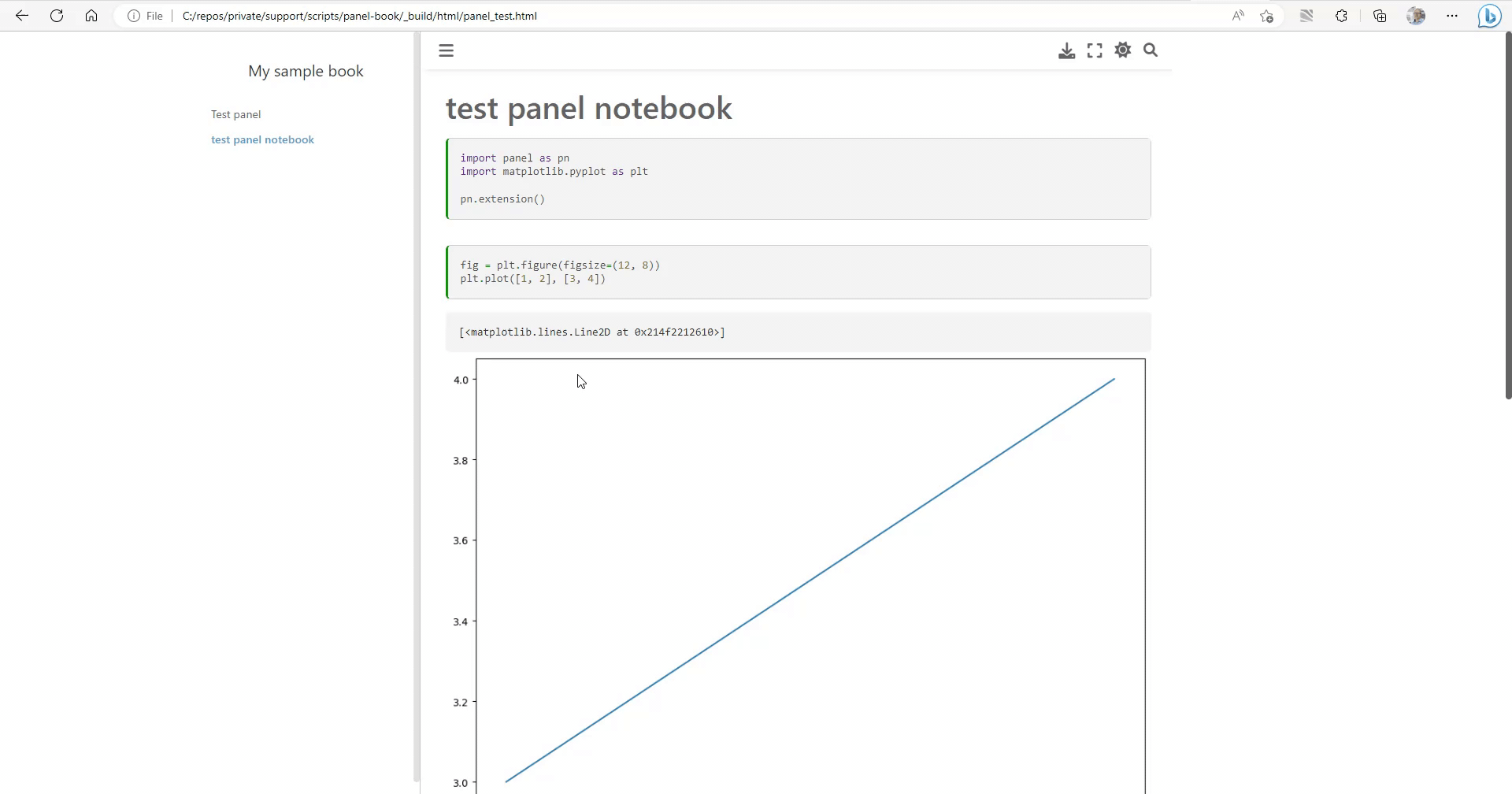# External non-embedded images for panel + nbconvert to html

I would like to be able to create standalone html dashboards with panel and have all plots in external pngs (not embedded) so the html is a reasonable size. Is that possible? I have a mix of matplotilb and panel + matplotlib and am converting from markdown notebooks to html in the context of jupyterbook. This works for regular matplotlib cells but not panel. Any advice helpful, including where else to seek help.
A simple example below:

``````import panel as pn
import matplotlib.pyplot as plt

pn.extension()
``````
``````fig = plt.figure(figsize=(12, 8))
plt.plot([1, 2], [3, 4])
``````
``````tabs = pn.Tabs()

fig = plt.figure(figsize=(12, 8))
plt.plot([1, 2], [3, 4])
pfig = pn.pane.Matplotlib(fig, dpi=144, tight=True)
tabs.append(('test', pfig))
plt.close()

tabs.embed()
``````
1 Like

You can for example `.save` to `.html` files.

## Example

``````import panel as pn
from matplotlib import cm
from matplotlib.figure import Figure
import numpy as np

pn.extension()

tabs = pn.Tabs()

fig0 = Figure(figsize=(12, 8))
ax0 = fig0.subplots()
Y, X = np.mgrid[-3:3:100j, -3:3:100j]
U = -1 - X**2 + Y
V = 1 + X - Y**2
speed = np.sqrt(U*U + V*V)
strm = ax0.streamplot(X, Y, U, V, color=U, linewidth=2, cmap=cm.autumn)
fig0.colorbar(strm.lines)
pfig = pn.pane.Matplotlib(fig0, dpi=144, tight=True)
tabs.append(('test', pfig))

tabs.embed()
tabs.save("dashboard.html")
``````
``````python script.py
``````
``````python -m http.server
``````

Open http://localhost:8000/dashboard.html and it will show

Thanks @Marc this is helpful but i dont think it allows me to create an html for the entire notebook including other cells without panel components.

1 Like

Ok. Then I did not understand you question well enough.

Can you describe the steps you would do to turn your notebook into html?

I use jupyterbook which itself uses myst-nb. I realize this may not be a panel issue specifically or maybe is an issue with how panel and jupyterbook / myst-nb interact. Thanks so much for your responses and help.

Basic example:

_config.yml

``````title: My sample book
author: The Jupyter Book Community
execute:
execute_notebooks: force
``````

_toc.yml

``````format: jb-book
root: intro
chapters:
- file: panel_test
``````

intro.md

``````# Test panel
``````

panel_test.md with ``` escaped with \:

``````---
jupytext:
text_representation:
extension: .md
format_name: myst
format_version: 0.13
jupytext_version: 1.14.5
kernelspec:
display_name: Python 3 (ipykernel)
language: python
name: python3
---

# test panel notebook

\```{code-cell} ipython3

import panel as pn
import matplotlib.pyplot as plt

pn.extension()
\```

\```{code-cell} ipython3

fig = plt.figure(figsize=(12, 8))
plt.plot([1, 2], [3, 4])
\```

\```{code-cell} ipython3

tabs = pn.Tabs()

fig = plt.figure(figsize=(12, 8))
plt.plot([1, 2], [3, 4])
pfig = pn.pane.Matplotlib(fig, dpi=144, tight=True)
tabs.append(('test', pfig))
plt.close()

tabs.embed()
\```
``````

That is a great minimum, reproducible example. Makes it so easy to understand your context and try it out.

I just created a fresh environment

``````python -m venv .venv
``````

and activated it. Then I installed the dependencies (and a bit more)

``````pip install panel hvplot holoviews jupyter-book jupyterlab matplotlib
``````

Then I created a folder called `panel-book` containing your files. Then I build the book

``````jupyter-book build panel-book
``````

Finally I opened the `panel_test.html` file in my browser.

To me it looks like below. I think it works fine for me?What would you expect?

It works for me also but for the straight matplotlib cell, the plot image gets saved to a png and not as an encoded string in the html. The panel tab images get stored in the html and if you have a lot of tabs then the html gets very large. Is that the case for your run above? Im hoping for a solution that always stores images external to the html.